# Show that the Matrix a is Unitary Where a = [ α + I γ − β + L β + L α − I γ ] is Unitary If α 2 + β 2 + γ 2 + ∂ 2 = 1 - Applied Mathematics 1

Show that the matrix A is unitary where A = [[alpha+igamma,-beta+idel],[beta+idel,alpha-igamma]] is unitary if alpha^2+beta^2+gamma^2+del^2=1

#### Solution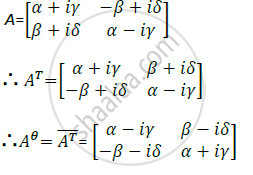Given, A is Unitary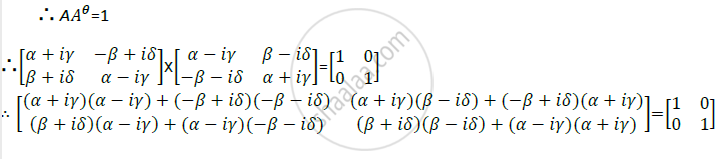Consider,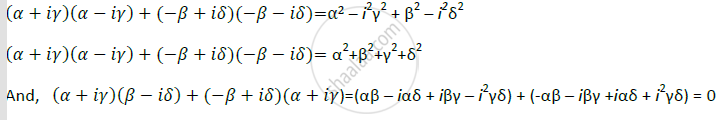Similarlly,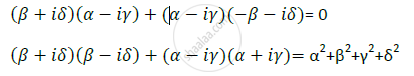Substituting (2),(3),(4)&(5) in (1),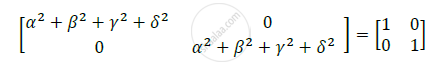Comparing corresponding terms, we get,

unitary if alpha^2+beta^2+gamma^2+del^2=1

Concept: Inverse of a Matrix
Is there an error in this question or solution?